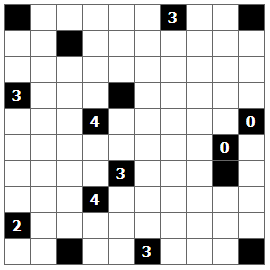# Shakashaka

﻿﻿﻿﻿﻿﻿﻿﻿
Translate this site.

Shakashaka (Proof of Quilt) is a logic puzzle with simple rules and challenging solutions.

The rules are simple. Shakashaka is played on a rectangular grid. The grid has both black cells and white cells in it.
The objective is to place black triangles in the white cell in such a way so that they form white rectangular (or square) areas.
- The triangles are right angled and occupy half of the white square divided diagonally.
- You can place triangles only in white cells
- The numbers in the black cells indicate how many triangles are adjacent, vertically and horizontally.
- The white rectangles can be either straight or rotated at 45°

Video Tutorial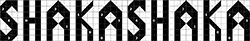Q. What am I supposed to do? :) A.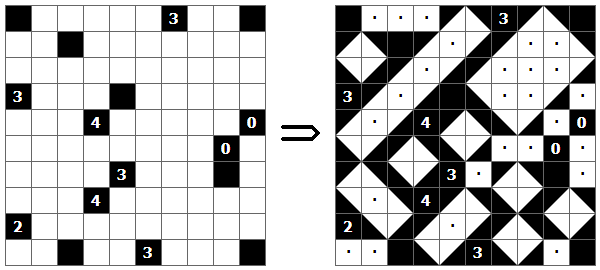Note - there are only perfect rectangular (or square) white shapes in the solution. Q. What are these numbers for? A. Each number indicates exactly how many triangles should be drawn around it. Black cells which do not have numbers inside can be surrounded by any number of triangles. Q. What is not allowed? A.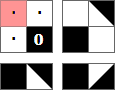These are all cases where it will be impossible to form a rectangular white shape. Q. Where do I start? A. Think logical. As an example take a look at this puzzle: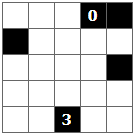Note the 3 on the bottom edge - it only has 3 cells around it so all of them should contain a triangle. 2 of those triangles can be placed in only one way.  You can also mark with dots the cells which don't contain a triangle for sure - in this case on the sides of the 0.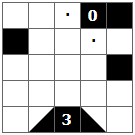When you place a triangle you can be sure that it is a part of an edge of a rectangle. It can either continue in the same direction or turn at 90°. A simple way of thinking is that if the edge bounces when it hits an obstacle: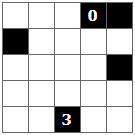Note the 0 and the cells which we already marked as white. The only way to place a triangle between them is this: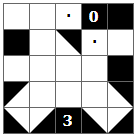Each edge of a rectengle should have an opposite one like this: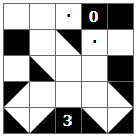In time you will discover your own patterns and strategies which makes solving shakashaka fun.